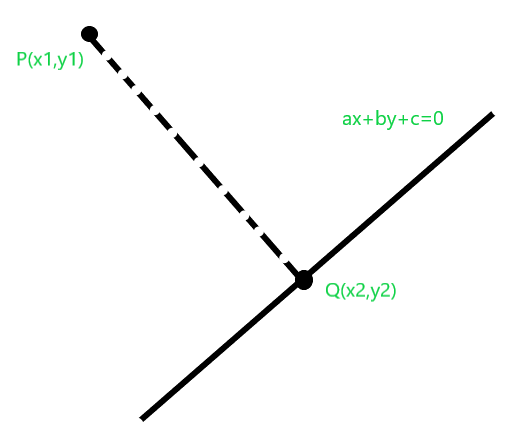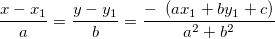Related Articles
Find foot of perpendicular from a point in 2 D plane to a Line
• Last Updated : 26 Mar, 2019

Given a point P in 2-D plane and equation of a line, the task is to find the foot of the perpendicular from P to the line.

Note: Equation of line is in form ax+by+c=0.

Examples:

```Input :  P=(1, 0), a = -1, b = 1, c = 0
Output : Q = (0.5, 0.5)
The foot of perpendicular from point (1, 0)
to line -x + y = 0 is (0.5, 0.5)

Input :  P=(3, 3), a = 0, b = 1, c = -2
Output : Q = (3, 2)
The foot of perpendicular from point (3, 3)
to line y-2 = 0 is (3, 2)
```

## Recommended: Please try your approach on {IDE} first, before moving on to the solution.Since equation of the line is given to be of the form ax + by + c = 0. Equation of line passing through P and is perpendicular to line. Therefore equation of line passing through P and Q becomes ay – bx + d = 0. Also P passes through line passing through P and Q, so we put coordinate of P in the above equation:

```ay1 - bx1 + d = 0
or, d = bx1 - ay1
```

Also, Q is the intersection of the given line and the line passing through P and Q. So we can find the solution of:

```ax + by + c = 0
and,
ay - bx + (bx1-ay1) = 0
```

Since a, b, c, d all are known we can find x and y here as:Below is the implementataion of the above approach:

## C++

 `// C++ program for implementation of ` `// the above approach ` `#include ` `using` `namespace` `std; ` ` `  `// Function to find foot of perpendicular from ` `// a point in 2 D plane to a Line ` `pair<``double``, ``double``> findFoot(``double` `a, ``double` `b, ``double` `c, ` `                              ``double` `x1, ``double` `y1) ` `{ ` `    ``double` `temp = -1 * (a * x1 + b * y1 + c) / (a * a + b * b); ` `    ``double` `x = temp * a + x1; ` `    ``double` `y = temp * b + y1; ` `    ``return` `make_pair(x, y); ` `} ` ` `  `// Driver Code ` `int` `main() ` `{ ` `    ``// Equation of line is ` `    ``// ax + by + c = 0 ` `    ``double` `a = 0.0; ` `    ``double` `b = 1.0; ` `    ``double` `c = -2; ` ` `  `    ``// Coordinates of point p(x1, y1). ` `    ``double` `x1 = 3.0; ` `    ``double` `y1 = 3.0; ` ` `  `    ``pair<``double``, ``double``> foot = findFoot(a, b, c, x1, y1); ` `    ``cout << foot.first << ``" "` `<< foot.second; ` ` `  `    ``return` `0; ` `} `

## Java

 `import` `javafx.util.Pair; ` ` `  `// Java program for implementation of ` `// the above approach ` `class` `GFG  ` `{ ` ` `  `// Function to find foot of perpendicular from ` `// a point in 2 D plane to a Line ` `static` `Pair findFoot(``double` `a, ``double` `b, ``double` `c, ` `                            ``double` `x1, ``double` `y1) ` `{ ` `    ``double` `temp = -``1` `* (a * x1 + b * y1 + c) / (a * a + b * b); ` `    ``double` `x = temp * a + x1; ` `    ``double` `y = temp * b + y1; ` `    ``return` `new` `Pair(x, y); ` `} ` ` `  `// Driver Code ` `public` `static` `void` `main(String[] args)  ` `{ ` `    ``// Equation of line is ` `    ``// ax + by + c = 0 ` `    ``double` `a = ``0.0``; ` `    ``double` `b = ``1.0``; ` `    ``double` `c = -``2``; ` ` `  `    ``// Coordinates of point p(x1, y1). ` `    ``double` `x1 = ``3.0``; ` `    ``double` `y1 = ``3.0``; ` ` `  `    ``Pair foot = findFoot(a, b, c, x1, y1); ` `    ``System.out.println(foot.getKey() + ``" "` `+ foot.getValue()); ` `    ``} ` `} ` ` `  `// This code contributed by Rajput-Ji `

## Python3

 `# Python3 implementation of the approach  ` ` `  `# Function to find foot of perpendicular  ` `# from a point in 2 D plane to a Line  ` `def` `findFoot(a, b, c, x1, y1):  ` ` `  `    ``temp ``=` `(``-``1` `*` `(a ``*` `x1 ``+` `b ``*` `y1 ``+` `c) ``/``/` `                  ``(a ``*` `a ``+` `b ``*` `b))  ` `    ``x ``=` `temp ``*` `a ``+` `x1  ` `    ``y ``=` `temp ``*` `b ``+` `y1  ` `    ``return` `(x, y)  ` ` `  `# Driver Code  ` `if` `__name__ ``=``=` `"__main__"``: ` ` `  `    ``# Equation of line is  ` `    ``# ax + by + c = 0  ` `    ``a, b, c ``=` `0.0``, ``1.0``, ``-``2` `     `  `    ``# Coordinates of point p(x1, y1).  ` `    ``x1, y1 ``=` `3.0``, ``3.0` ` `  `    ``foot ``=` `findFoot(a, b, c, x1, y1)  ` `    ``print``(``int``(foot[``0``]), ``int``(foot[``1``]))  ` `         `  `# This code is contributed ` `# by Rituraj Jain `

## C#

 `// C# program for implementation of  ` `// the above approach  ` `using` `System; ` ` `  `class` `GFG  ` `{  ` `    ``// Pair class ` `    ``public` `class` `Pair ` `    ``{ ` `        ``public` `double` `first,second; ` `        ``public` `Pair(``double` `a,``double` `b) ` `        ``{ ` `            ``first = a; ` `            ``second = b; ` `        ``} ` `    ``} ` ` `  `// Function to find foot of perpendicular from  ` `// a point in 2 D plane to a Line  ` `static` `Pair findFoot(``double` `a, ``double` `b, ``double` `c,  ` `                            ``double` `x1, ``double` `y1)  ` `{  ` `    ``double` `temp = -1 * (a * x1 + b * y1 + c) / (a * a + b * b);  ` `    ``double` `x = temp * a + x1;  ` `    ``double` `y = temp * b + y1;  ` `    ``return` `new` `Pair(x, y);  ` `}  ` ` `  `// Driver Code  ` `public` `static` `void` `Main(String []args)  ` `{  ` `    ``// Equation of line is  ` `    ``// ax + by + c = 0  ` `    ``double` `a = 0.0;  ` `    ``double` `b = 1.0;  ` `    ``double` `c = -2;  ` ` `  `    ``// Coordinates of point p(x1, y1).  ` `    ``double` `x1 = 3.0;  ` `    ``double` `y1 = 3.0;  ` ` `  `    ``Pair foot = findFoot(a, b, c, x1, y1);  ` `    ``Console.WriteLine(foot.first + ``" "` `+ foot.second);  ` `    ``}  ` `}  ` ` `  `// This code contributed by Arnab Kundu `

## PHP

 ` `

Output:

```3 2
```

Attention reader! Don’t stop learning now. Get hold of all the important DSA concepts with the DSA Self Paced Course at a student-friendly price and become industry ready.

My Personal Notes arrow_drop_up
Recommended Articles
Page :